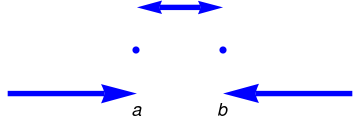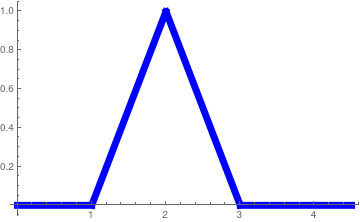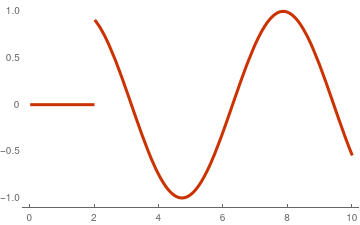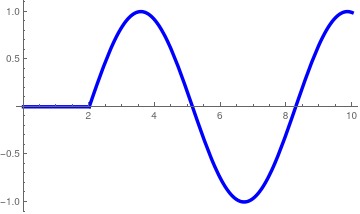# Laplace transform of intermittent functions

First, we remind the definition of a piecewise continuous function. For our applications, we don't need the general definition of such function made previously. Instead, we restrict ourself with the following simplified version. A function f(t), defined on semi-infinite interval [0, ∞), is called piecewise continuous or intermittent if this interval can be broken into finite number of subintervals

$[0, \infty ) = [0, b_1) \cup (a_2 , b_2 ) \cup \cdots \cup (a_m, \infty ) ,$
so that the function f(t) is continuous on each of them and has finite limit values at the endpoints. A piecewise continuous function can be defined as
\begin{equation} \label{EqInput.1} f(t) = \begin{cases} f_1 (t) , & \ 0 < t < b_1 , \\ f_2 (t) , & \ a_2 < t < b_2 , \\ \vdots \\ f_m (t) , & a_m < t < \infty , \end{cases} \end{equation}
where each function fk(t), k = 1, 2, … , m, is continuous on the interval (𝑎k, bk) and has finite limit values at 𝑎k and bk. Since we are going to apply the Laplace transformation to these intermittent functions, we require that the function fm(t) grows no faster than exponential function at infinity in order to define its Laplace transform:

\begin{equation} \label{EqInput.2} f^L (\lambda ) = \left( {\cal L} \,f \right) (\lambda ) = \int_0^{\infty} f(t)\,e^{-\lambda t} \,{\text d} t . \end{equation}
In order for integral \eqref{EqInput.2} to converge, the function f(t) should grow at infinity no faster than exponential. There is known a very wide class of functions for which we know for sure that their Laplace transformations converge. It is referred to as functions-original, and they were discussed in the first section.

We usually do not specify the values of the piecewise continuous functions at the points of discontinuity (if any) because they do not effect the value of Laplace's integral \eqref{EqInput.2}. However, the inverse Laplace transformation always defines the value of the function at the point of discontinuity to be the mean value of its left and right limit values.

${\cal L}^{-1} \left[ f^L (\lambda ) \right] (t) = \frac{f(t^{-}) + f(t^{+})}{2} = \frac{f(t-0) + f(t+0)}{2} = \lim_{\varepsilon \to 0, \ \varepsilon > 0} \, \frac{f(t- \varepsilon ) + f(t+ \varepsilon )}{2} = \lim_{\varepsilon \downarrow 0} \, \frac{f(t- \varepsilon ) + f(t+ \varepsilon )}{2} .$

The key to handle the Laplace transformation of intermittent functions lies in a notational one. We need a way to write a piecewise continuous function as simple formula so that it may be handled in convenient manner. This involves the unit step or Heaviside function:

\begin{equation} \label{EqInput.3} H(t) = \begin{cases} 1 , & \ \mbox{ for }\ t > 0, \\ 1/2, & \ \mbox{ for }\ t=0, \\ 0, & \ \mbox{ for }\ t < 0. \end{cases} \end{equation}
For the Laplace transformation \eqref{EqInput.2}, it does not matter what is the value of the Heaviside function at t = 0. You can define the unit step function as
$u(t) = \begin{cases} 1 , & \ \mbox{ for }\ t > 0, \\ \mbox{whatever you want}, & \ \mbox{ for }\ t=0, \\ 0, & \ \mbox{ for }\ t < 0. \end{cases}$
Then its Laplace transform is
${\cal L} \left[ u(t) \right] = {\cal L} \left[ H(t) \right] = \int_0^{\infty} e^{-\lambda t} {\text d} t = \frac{1}{\lambda} ,$
independently on the value of these functions at t = 0. However, the inverse Laplace transform restores only the Heaviside function, but not u(t). This is the reason why we utilize the definition of the Heaviside function, but not any other unit step function---we need a one-to-one correspondence.

${\cal L}^{-1} \left[ \frac{1}{\lambda} \right] = H(t) .$

The shifted Heaviside function H(t−c) can be thought of as an “on”/“off” switch with a trigger value c. If we look to the left of c, the function evaluates to zero (the “off” state), and if we look to the right of c, the function evaluates to one (the “on” state).

The importance of the Heaviside function lies in the fact that it can be combined with itself and other functions to generalize the notion of turn functions “on” or “off” over certain regions of t. In particular, if we a given two positive numbers 0 < 𝑎 < b, we can define the window function

\begin{equation} \label{EqInput.4} W(t; a, b) = H (t-a) - H(t-b) = \begin{cases} 0 , & \ \mbox{ for }\ t < a, \\ 1, & \ \mbox{ for }\ a < t < b, \\ 0, & \ \mbox{ for }\ t > b. \end{cases} \end{equation}We plot the window: a1 = Graphics[{Arrowheads[0.1], Thickness[0.015], Blue, Arrow[{{-0.5, 0}, {1, 0}}]}]; a2 = Graphics[{Arrowheads[{-0.07, 0.07}], Thickness[0.015], Blue, Arrow[{{1, 1}, {2, 1}}]}]; a3 = Graphics[{Arrowheads[0.1], Thickness[0.015], Blue, Arrow[{{3.5, 0}, {2, 0}}]}]; p1 = Graphics[{PointSize[0.02], Blue, Point[{1, 1/2}]}]; p2 = Graphics[{PointSize[0.02], Blue, Point[{2, 1/2}]}]; t1 = Graphics[{Black, Text[Style["a", Italic, 18], {1, -0.2}]}]; t2 = Graphics[{Black, Text[Style["b", Italic, 18], {2, -0.2}]}]; Show[a1, a2, a3, p1, p2, t1, t2] Window plot. Mathematica code

In other words, we are only in the “on” state in the region 𝑎 ≤ tb; otherwise, we are “off”. So this form allows us to define intervals that are “on”. Of course, what we are interested in turning “off” and “on” is not simply the value one. Rather, if we are giving some function g(t) on the interval [𝑎, b], then we are manipulating this function as
$g(t)\, W(t; a, b) = g(t) \left[ H(t-a) - H(t-b) \right] = \begin{cases} 0 , & \ \mbox{ for }\ t < a, \\ g(t), & \ \mbox{ for }\ a < t < b, \\ 0, & \ \mbox{ for }\ t > b. \end{cases}$
With window function, we can represent a piecewise continuous function as the sum:
\begin{equation} \label{EqInput.5} f(t) = f_1 (t)\,W\left( t; 0, b_1 \right) + f_2 (t)\, W\left( t; a_2, b_2 \right) + \cdots + f_m (t)\,,W\left( t; a_m , \infty \right) . \end{equation}
The problem of finding the Laplace transformation of the piecewise continuous function \eqref{EqInput.5} is reduced to determination of the Laplace transform of every term in this series. Since the Laplace transform of the window function is known
\begin{equation} \label{EqInput.6} {\cal L} \left[ W(t; a,b) \right] = {\cal L} \left[ H(t-a) - H(t-b) \right] = \frac{1}{\lambda} \, e^{-a\lambda} - \frac{1}{\lambda} \, e^{-b\lambda} , \end{equation}
we need to apply the shift property of the Laplace transform.
Theorem [Shift Rule]: Let $${\cal L}\left[ g(t) \right] = g^L (\lambda )$$ be the Laplace transform of function g. Then
\begin{equation} \label{EqInput.7} {\cal L} \left[ H(t-a)\,g(t-a) \right] = e^{-a\lambda} g^L (\lambda ) , \qquad a> 0; \end{equation}
and, conversely,
\begin{equation} \label{EqInput.8} {\cal L}^{-1} \left[ e^{-a\lambda} g^L (\lambda ) \right] = H(t-a)\,g(t-a) , \qquad a> 0. \end{equation}

The Laplace transformation exists for many functions of a positive real variable (usually associated with time) including discontinuous functions. Of course, the Laplace transform does not exist for arbitrary functions, but only for those that belong to special classes. Previously, we identified that the Laplace transform exists for functions with finite jumps and that grow no faster than an exponential function at infinity. The algorithm finding a Laplace transform of an intermittent function consists of two steps:

1. Rewrite the given piecewise continuous function through shifted Heaviside functions.
2. Use the shift rule $${\cal L} \left[ H(t-a)\, f(t-a) \right] = e^{a\lambda}\, {\cal L} \left[ f(t) \right] .$$
We demonstrate this approach in numerous examples.
Example 1: Consider the following continuous function
$f(t) = \begin{cases} 0, & \ 0 < t < 1 , \\ t-1, & \ 1 < t < 2 , \\ 3-t , & \ 2 < t < 3 , \\ 0 , & \ t> 3, \end{cases} \tag{1.1}$
which is commonly called a “tent” function. This could model, for instance, an external signal that begins to climb at t = 1, then begins to fall at t = 2, and eventually reaches zero (i.e. no signal) at t = 3.We plot the tent function: Plot[Piecewise[{{0, 0 < t < 1}, {t - 1, 1 < t < 2}, {3 - t, 2 < t < 3}}], {t, 0, 4.5}, PlotStyle -> {Thickness[0.02], Blue}] Tent function. Mathematica code

To find the Laplace transform of function (1.1), we represent it as the sume of functions involving the window function:
$f(t) = \left( t-1 \right) \left[ H(t-1) - H(t-2) \right] + \left( 3-t \right) \left[ H(t-2) - H(t-3) \right]$
In order to apply the shift rule, we rewrite every term in appropriate way:
\begin{align*} \left( t-1 \right) H(t-2) &= \left( t-2 +1 \right) H(t-2) = \left( t-2 \right) H(t-2) + H(t-2) , \\ \left( 3-t \right) H(t-2) &= \left( 1 + 2-t \right) H(t-2) = H(t-2) - \left( t-2 \right) H(t-2) . \end{align*}
Then
$f(t) = \left( t-1 \right) H(t-1) - 2 \left( t-2 \right) H(t-2) + \left( t-3 \right) H(t-3) .$
Now applying the Laplace transform and using shift rule \eqref{EqInput.7}, we get
$f^L (\lambda ) = \frac{1}{\lambda^2} \left[ e^{-\lambda} - 2\,e^{-2\lambda} + e^{-3\lambda} \right] .$
We check our formula with Mathematica:
Simplify[Integrate[(t - 1)*Exp[-s*t], {t, 1, 2}] + Integrate[(3 - t)*Exp[-s*t], {t, 2, 3}]]
(E^(-3 s) (-1 + E^s)^2)/s^2
■

Example 2: We consider two simple piecewise functions

$f(x) = \begin{cases} t^2 , & \ \mbox{ when }\ 3< t < 5, \\ 0, & \ \mbox{ otherwise}, \end{cases} \qquad \mbox{and} \qquad g(x) = \begin{cases} t^3 , & \ \mbox{ when }\ 2< t < 4, \\ 0, & \ \mbox{ otherwise}. \end{cases}$
First, we rewrite these functions using the shifted Heaviside function as follows:
\begin{align*} f(t) &= t^2 \left[ H(t-3) - H(t-5) \right] = t^2 H(t-3) - t^2 H(t-5) , \\ g(t) &= t^3 \left[ H(t-2) - H(t-4) \right] = t^3 H(t-2) - t^3 H(t-4) , \end{align*}
Each of components can be transformed as
\begin{align*} t^2 H(t-3) &= \left( t-3 + 3 \right)^2 H(t-3) = \left[ (t-3)^2 + 2\,(t-3)\,3 + 3^2 \right] H(t-3) , \\ t^2 H(t-5) &= \left( t-5 + 5 \right)^2 H(t-5) = \left[ (t-5)^2 + 2\,(t-5)\,5 + 5^2 \right] H(t-5) , \\ t^3 H(t-2) &= \left( t-2 + 2 \right)^3 H(t-2) = \left[ (t-2)^3 + 3\,(t-2)^2 2 + 3\,(t-2)\,2^2 + 2^3 \right] H(t-2) , \\ t^3 H(t-4) &= \left( t-4 + 4 \right)^3 H(t-4) = \left[ (t-4)^3 + 3\,(t-2)^2 4 + 3\,(t-2)\,4^2 + 4^3 \right] H(t-4) , \end{align*}
because
$(t+a)^2 = t^2 + 2a\,t + a^2 ,\qquad (t+a)^3 = t^3 + 3\,t^2 a + 3\,t\,a^2 + a^3 .$
Now we prepare the functions in the form applicable to the Laplace shift rule:
\begin{align*} f(t) &= \left[ (t-3)^2 + 2\,(t-3)\,3 + 3^2 \right] H(t-3) - \left[ (t-5)^2 + 2\,(t-5)\,5 + 5^2 \right] H(t-5) , \\ g(t) &= \left[ (t-2)^3 + 3\,(t-2)^2 2 + 3\,(t-2)\,2^2 + 2^3 \right] H(t-2) -\left[ (t-4)^3 + 3\,(t-4)^2 4 + 3\,(t-4)\,4^2 + 4^3 \right] H(t-4) , \end{align*}
because every term is of the form f(t-a) H(t-a), where H(t) is the Heaviside unit function. The Laplace transforms of these functions follow
\begin{align*} {\cal L} \left[ f(t) \right] (\lambda ) &= \left[ \frac{2}{\lambda^3} + \frac{6}{\lambda^2} + \frac{9}{\lambda} \right] e^{-3\lambda} - \left[ \frac{2}{\lambda^3} + \frac{10}{\lambda^2} + \frac{25}{\lambda} \right] e^{-5\lambda} , \\ {\cal L} \left[ g(t) \right] (\lambda ) &= \left[ \frac{3!}{\lambda^4} + \frac{12}{\lambda^3} + \frac{12}{\lambda^2} + \frac{8}{\lambda} \right] e^{-2\lambda} - \left[\frac{3!}{\lambda^4} + \frac{24}{\lambda^3} + \frac{48}{\lambda^2} + \frac{64}{\lambda} \right] e^{-4\lambda} . \end{align*}
We check the answers with Mathematica:
Integrate[ t^2 *Exp[-s*t], {t, 3, 5}]
(E^(-5 s) (-2 - 10 s - 25 s^2 + E^(2 s) (2 + 6 s + 9 s^2)))/s^3
and
Integrate[ t^3 *Exp[-s*t], {t, 2, 4}]
(E^(-4 s) (-6 - 8 s (3 + 6 s + 8 s^2) + 2 E^(2 s) (3 + 2 s (3 + s (3 + 2 s)))))/s^4
■
Example 3: Consider the following continuous function
$f(t) = \begin{cases} t^2, & \ 0 < t < 1 , \\ t , & \ 1 < t < 2 , \\ 1 , & \ t> 2, \end{cases} \tag{3.1}$
We rewrite the given function using the window functions:
$f(t) = t^2 \left[ H(t) - H(t-1) \right] + t \left[ H(t-1) - H(t-2) \right] + H(t-2) .$
Transferring every term in the sum above into the form suitable for application of the shift rule, we get
\begin{align*} -t^2 H(t-1) &= - \left( t-1 + 1 \right)^2 H(t-1) = -\left[ (t-1)^2 + 2\,(t-1) + 1 \right] H(t-1) , \\ t\,H(t-1) &= \left( t-1+1 \right) H(t-1) , \\ -t\,H(t-2) &= - \left( t-2+2 \right) H(t-2) \end{align*}
This allows us to write the given piecewise function as the sum of shifted functions:
$f(t) = t^2 H(t) - \left[ (t-1)^2 +(t-1) \right] H(t-1) - \left[ t-2 +1 \right] H(t-2) .$
Now we apply the Laplace transform to each term using the shift rule and obtain
$f^L (\lambda ) = \frac{2}{\lambda^3} - \frac{2}{\lambda^3}\, e^{-\lambda} - \frac{1}{\lambda^2}\,e^{-\lambda} - \frac{1}{\lambda^2}\,e^{-2\lambda} - \frac{1}{\lambda}\,e^{-2\lambda} .$
We check the answer with Mathematica:
Assuming[s > 0, Simplify[Integrate[t^2*Exp[-s*t], {t, 0, 1}] + Integrate[t*Exp[-s*t], {t, 1, 2}] + Integrate[Exp[-s*t], {t, 2, Infinity}] ]]
(E^(-2 s) (2 E^(2 s) - s (1 + s) - E^s (2 + s)))/s^3
■

Example 4: Consider a piecewise continuous function

$f(t) = \begin{cases} 2, & \quad 0 < t < 1 , \\ t-3, & \quad 1 < t < 3 , \\ t^2 , & \quad t > 3 . \end{cases}$
We rewrite the given function using our lovely Heaviside function:
\begin{eqnarray*} f(t) &=& 2 \left[ H(t) - H(t-1) \right] + (t-3) \left[ H(t-1) - H(t-3) \right] + t^2 \, H(t-3) \\ &=& 2\, H(t) + (t-5)\, H(t-1) + \left[ t^2 - (t-3) \right] H(t-3) \\ &=& 2\, H(t) + (t-1-4)\, H(t-1) + \left[ (t-3)^2 +5\, (t-3) +9 \right] H(t-3) . \end{eqnarray*}
Now we are ready to apply the shift rule to obtain
${\cal L} \left[ f(t) \right] (\lambda ) = f^L (\lambda ) = \frac{2}{\lambda} + \left( \frac{1}{\lambda^2} - \frac{4}{\lambda} \right) e^{-\lambda} + \left( \frac{2}{\lambda^3} + \frac{5}{\lambda^2} + \frac{9}{\lambda} \right) e^{-2\lambda} .$
We check our answer with Mathematica:
Integrate[2*Exp[-s*t], {t, 0, 1}] + Integrate[(t - 3)*Exp[-s*t], {t, 1, 3}] + Integrate[t^2*Exp[-s*t], {t, 3, Infinity}]
■

Example 5: Consider another piecewise continuous function

$g(t) = \begin{cases} t, & \quad 0 < t < 1 , \\ (t-2)^2, & \quad 1 < t < 2 , \\ 6-t^2 , & \quad t > 2 . \end{cases}$
We rewrite the given function using our lovely Heaviside function:
\begin{eqnarray*} g(t) &=& t \left[ H(t) - H(t-1) \right] + (t-2)^2 \left[ H(t-1) - H(t-2) \right] + \left( 6 - t^2 \right) H(t-2) \\ &=& t\, H(t) - (t-1+1)\, H(t-1) + \left( t-1-1 \right)^2 H(t-1) - (t-2)^2 H(t-2) + \left[ 6 - (t-2+2)^2 \right] H(t-2) \\ &=& t\, H(t) + \left[ (t-1)^2 -3(t-1)\right] H(t-1) + \left[ 2- 4(t-2) -2 (t-2)^2 \right] H(t-2) . \end{eqnarray*}
Now we are ready to apply the shift rule to obtain
${\cal L} \left[ g(t) \right] (\lambda ) = g^L (\lambda ) = \frac{1}{\lambda^2} + \left( \frac{2}{\lambda^3} - \frac{3}{\lambda^2} \right) e^{-\lambda} + \left( \frac{2}{\lambda} - \frac{4}{\lambda^2} - \frac{4}{\lambda^3} \right) e^{-3\lambda} .$
We check our answer with Mathematica:
g[t_] = Piecewise[{{t, 0 < t < 1}, {(t - 2)^2, 1 < t < 2}, {6 - t^2, 2 < t}}]
LaplaceTransform[g[t], t, lambda]
Out= (1/(lambda^3))E^(-2 lambda) (-4 - 4 lambda - E^lambda lambda + E^(2 lambda) lambda + 2 lambda^2 - E^lambda lambda^2 + Gamma[3, -lambda])
Or integrate directly:
Integrate[t*Exp[-s*t], {t, 0, 1}] + Integrate[(t - 2)^2 *Exp[-s*t], {t, 1, 2}] + Integrate[(6 - t^2)*Exp[-s*t], {t, 2, Infinity}]
Simplify[%]
ConditionalExpression[( E^(-2 s) (E^s (2 - 3 s) + E^(2 s) s + 2 (-2 - 2 s + s^2)))/s^3, Re[s] > 0]
■

Example 6: We consider two functions

$f(t) = \sin t\,H(t-2) = \begin{cases} 0. & \ \mbox{ if} \quad t < 2, \\ \sin t , & \ \mbox{ if} \quad t > 2; \end{cases} \qquad g(t) = \sin (t-2)\,H(t-2) = \begin{cases} 0. & \ \mbox{ if} \quad t < 2, \\ \sin t , & \ \mbox{ if} \quad t > 2; \end{cases}$
We plot these functions with Mathematica.
f[t_] = Sin[t]*HeavisideTheta[t-2];
g[t_] = Sin[t-2]*HeavisideTheta[t-2];
Plot[f[t], {t, 0, 10}, PlotTheme -> "Web"]
Plot[g[t], {t, 0, 10}, PlotStyle -> {Blue, Thickness[0.01]}]sin(t) H(t−2) sin(t−2) H(t−2)

Mathematica handles the Shift Rule:

LaplaceTransform[UnitStep[t - 2]*Sin[t], t, lambda]
Out= (E^(-2 lambda) (Cos + lambda Sin))/(1 + lambda^2)
${\cal L}_{t\to\lambda} \left[ \sin t\,H(t-2) \right] = \frac{cos 2 + \lambda\,\sin 2}{1 + \lambda^2} \, e^{-2\lambda} .$
LaplaceTransform[UnitStep[t - 2]*Sin[t - 2], t, lambda]
Out= E^(-2 lambda)/(1 + lambda^2)
${\cal L}_{t\to\lambda} \left[ \sin (t-2)\,H(t-2) \right] = \frac{1}{1 + \lambda^2} \, e^{-2\lambda} .$

Finally, we find the Laplace transforms of the Dirac delta function:

${\cal L}_{t\to \lambda} \left[ \delta (-2)\,\sin t \right] = \sin 2\, e^{-2\lambda} , \qquad {\cal L}_{t\to \lambda} \left[ \delta (-2)\,\sin (t-2) \right] = 0 .$
LaplaceTransform[DiracDelta[t - 2]*Sin[t - 2], t, lambda]
Out= 0
LaplaceTransform[DiracDelta[t - 2]*Sin[t], t, lambda]
Out= E^(-2 lambda) Sin

■

Example 7: Consider a piecewise continuous function

$f(t) = \begin{cases} 0, & \ \mbox{ for }\ 0 < t < \pi , \\ \sin (t), & \ \mbox{ for }\ \pi < t < 2\pi , \\ 0, & \ \mbox{ for }\ 2\pi < t < 5\pi /2 , \\ \sin (t), & \ \mbox{ for }\ 5\pi /2 < t < 4\pi . \end{cases}$
First, we rewrite the function using the Heaviside function
$f(t) = \sin (t) \left[ H(t-\pi ) - H(t- 2\pi ) + H(t-5\pi/2 ) - H(t- 4\pi ) \right] ,$
which we rewrite as
$f(t) = -\sin (t-\pi ) \,H(t-\pi ) - \sin (t-2\pi ) \,H(t-2\pi ) + \cos (t - 5\pi /2) - \sin (t-4\pi ) \,H(t-4\pi ) .$
Now we are ready to apply the shift rule to obtain the Laplace transform of the given function
$f^L (\lambda ) = - \frac{1}{\lambda^2 + 1}\, e^{-\pi\lambda} - \frac{1}{\lambda^2 + 1}\, e^{-2\pi\lambda} + \frac{\lambda}{\lambda^2 + 1}\, e^{-5\pi\lambda /2} - \frac{1}{\lambda^2 + 1}\, e^{-4\pi\lambda} .$
Finally, we check with Mathematica:
Integrate[Sin[t]*Exp[-s*t], {t, Pi, 2*Pi}] + Integrate[Sin[t]*Exp[-s*t], {t, 5*Pi/2, 4*Pi}]
-((E^(-2 $Pi] s) (1 + E^(\[Pi] s)))/(1 + s^2)) + ( E^(-4 \[Pi] s) (-1 + E^((3 \[Pi] s)/2) s))/(1 + s^2) ■ Example 8: Consider a piecewise continuous function \[ f(t) = \begin{cases} 1, & \ \mbox{ for }\ 0 < t < 2 , \\ e^{-3(t-5)}, & \ \mbox{ for }\ 2 < t < \infty . \end{cases}$
First, we rewrite the function using the Heaviside function
$f(t) = H(t) - H(t-2) + e^{-3(t-5)} H(t-2) ,$
To use the shift rule, we rewrite the exponential function in the form that contains only argument t-2:
$e^{-3(t-5)} = e^{-3(t- 2 -3)} = e^{-3(t- 2) + 9} = e^9 e^{-3(t- 2)} .$
Now we are ready to apply the Laplace transform
${\cal L} \left[ f \right] (\lambda ) = \frac{1}{\lambda} - \frac{1}{\lambda} \,e^{-2\lambda} + e^9 \,\frac{1}{\lambda +3}\,e^{-2\lambda}$
because the Laplace transform of the exponential function is $${\cal L} \left[ e^{-at} \right] = \left( \lambda + a \right)^{-1} .$$    ■